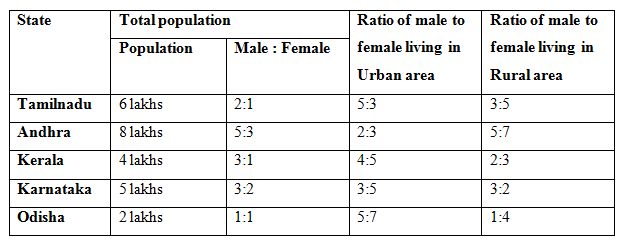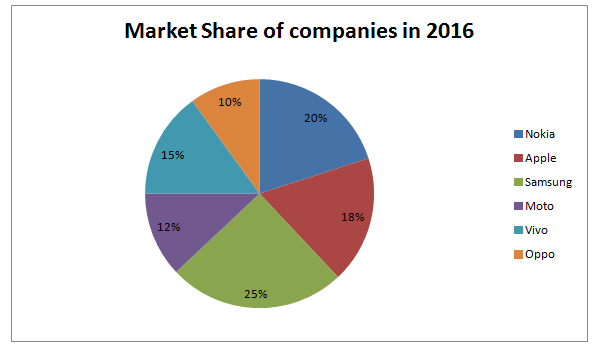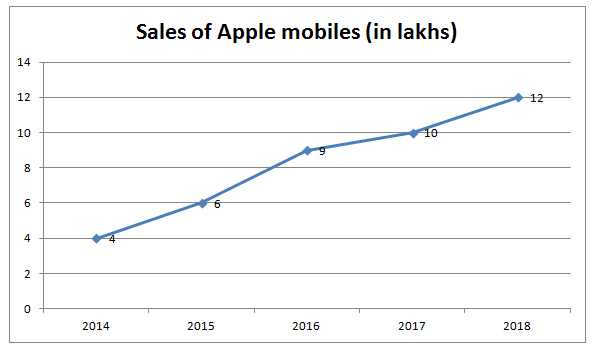# SBI PO Quantitative Aptitude Questions 2019 (Day-6) High Level New Pattern

SBI PO 2019 Notification is about to come and it is the most awaited exam among the aspirants. We all know that new pattern questions are introducing every year in the SBI PO exam. Further, the questions are getting tougher and beyond the level of the candidate’s expectations.

Our IBPS Guide is providing High-Level New Pattern Quantitative Aptitude Questions for SBI PO 2019 so the aspirants can practice it on a daily basis. These questions are framed by our skilled experts after understanding your needs thoroughly. Aspirants can practice these high-level questions daily to familiarize with the exact exam pattern. We wish that your rigorous preparation leads you to a successful target of becoming SBI PO.

#### “Be not afraid of growing slowly; be afraid only of standing still”

[WpProQuiz 4721]### Click Here for SBI PO Pre 2019 High-Quality Mocks Exactly on SBI Standard

Direction (1 – 5): Study the following information carefully and answer the given questions:1) What is the percentage of the population living in urban area having the age below 30 years out of the total population of Tamilnadu?

Statement I: If the age of 45% male and 50% female living in urban area of Tamilnadu is below 30 years.

Statement II: If the 60% of the total population of Tamilnadu living in urban area.

a) Only I

b) Only II

c) Either I or II sufficient

d) All I and II necessary to answer the question

e) The question can’t be answered even with all I and II

2) Quantity I: If 90% of the population of Kerala living in urban area, then what is the sum of the male population in rural area and the female population in urban area?

Quantity II: What is the difference between the female population of Karnataka and the female population of Andhra?

a) Quantity I > Quantity II

b) Quantity I ≥ Quantity II

c) Quantity II > Quantity I

d) Quantity II ≥ Quantity I

e) Quantity I = Quantity II or Relation cannot be established

3) Quantity I: The urban population of Andhra is 60 %. Out of the urban population of Andhra, 30% and 45% of the urban male and female is illiterate respectively. Then find the sum of the rural population of Andhra and the literate population of urban area?

Quantity II: What is the sum of the total population of Odisha and the female population of Karnataka?

a) Quantity I > Quantity II

b) Quantity I ≥ Quantity II

c) Quantity II > Quantity I

d) Quantity II ≥ Quantity I

e) Quantity I = Quantity II or Relation cannot be established

4) If 30%, 40%, 20%, 40% and 50% of the population lives in the rural area of Tamilnadu, Andhra, Kerala, Karnataka and Odisha respectively. Which of the following statement is correct?

Statement I: The urban male population of Tamilnadu is equal to the urban female population of Andhra.

Statement II: Rural female population of Karnataka is equal to the Rural female population of Odisha.

Statement III: Rural population of Karnataka is equal to the sum of the rural population of Tamilnadu and the rural male population of the Odisha together.

a) Only I

b) Only II

c) Only III

d) Both I and II

e) Both II and III5) What is the difference between total male population of all the states together and total of the female population of all the states together?

a) 6 lakhs

b) 7 lakhs

c) 9 lakhs

d) 5 lakhs

e) None of these

Direction (6 – 10): Study the following information carefully and answer the given questions:

The Pie-chart shows the market share of mobiles sold by different companies in the year 2016.The line graph shows the sales of Apple for the given period.6) Quantity I: If the market share of sales in 2017 is increased by 30% of the percentage share in 2016, then find the sale of Nokia in the year 2017, if the total value of all companies in 2017 is 60 lakhs?

Quantity II: If the market share of sales in 2014 is same as that in 2016, then find the sale of Moto in the year 2014?

a) Quantity I > Quantity II

b) Quantity I ≥ Quantity II

c) Quantity II > Quantity I

d) Quantity II ≥ Quantity I

e) Quantity I = Quantity II or Relation cannot be established

7) Quantity I: What is the average sale of Apple in the given period?

Quantity II: What is average sale of Samsung, Vivo and Oppo in 2016?

a) Quantity I > Quantity II

b) Quantity I ≥ Quantity II

c) Quantity II > Quantity I

d) Quantity II ≥ Quantity I

e) Quantity I = Quantity II or Relation cannot be established

8) If the market share of 2016 is equal to the market share of 2017.

Quantity I: What is the difference between the sale of Oppo and Nokia?

Quantity II: What is the difference between the sale of Samsung and Vivo?

a) Quantity I > Quantity II

b) Quantity I ≥ Quantity II

c) Quantity II > Quantity I

d) Quantity II ≥ Quantity I

e) Quantity I = Quantity II or Relation cannot be established

9) Quantity I: The sale of Apple in 2017 is what percent of the sale of Moto in 2016?

Quantity II: The sale of Samsung in 2016 is what percent of the sale of Nokia in 2016?

a) Quantity I > Quantity II

b) Quantity I ≥ Quantity II

c) Quantity II > Quantity I

d) Quantity II ≥ Quantity I

e) Quantity I = Quantity II or Relation cannot be established

10) Quantity I: What is the average sale of Nokia, Apple, Samsung, Moto, Vivo and Oppo in 2016?

Quantity II: If the market share of all the companies in 2018 is increases by 10% of the percentage share in 2016, then find the average sale of all the companies in 2018?

a) Quantity I > Quantity II

b) Quantity I ≥ Quantity II

c) Quantity II > Quantity I

d) Quantity II ≥ Quantity I

e) Quantity I = Quantity II or Relation cannot be established

Direction (1 – 5):

From Statement I,

Given data is not sufficient to the answer the question.

So, Statement I alone is not sufficient to the answer the question.

From Statement II,

Urban population = (60/100)*6 = 3.6 lakhs

Rural population = (40/100)*6 = 2.4 lakhs

So, Statement II alone is not sufficient to the answer the question.

From I and II,

Urban Male = (5/8)*3.6 lakhs = 2.25 lakhs

Urban female = (3/8)*3.6 lakhs = 1.35 lakhs

Below 30 male = (45/100)*2.25 lakhs = 101250

Below 30 female = (50/100)*1.35 lakhs = 67500

Total of below 30 = 101250 + 67500 = 168750

Required percentage = (168750/600000)*100 = 28.125%

From quantity I,

Urban population = (90/100)*4 lakhs = 3.6 lakhs

Female population in urban= (5/9)*3.6 = 2 lakhs

Rural population = (10/100)*4 lakhs = 40000

Male population in rural = (2/5)*40000 = 16000

Sum=200000 + 16000 = 216000 = 2.16 lakhs

From quantity II,

Karnataka female population = (2/5)*5=2 lakhs

Female population in Andhra = (3/8)*8=3 lakhs

Difference = 3 – 2 = 1 lakh

Quantity I > Quantity II

From quantity I,

Urban population of Andhra = (60/100)*8 = 4.8 lakhs

Urban male = (2/5)*4.8 = 1.92 lakhs

Literate male = (70/100)*1.92 lakhs = 1.344 lakhs

Urban female = (3/5)*4.8 = 2.88 lakhs

Literate female = (55/100)*2.88 = 1.584 lakhs

Literate urban = 1.344 + 1.584 = 2.928 lakhs

Rural = (40/100)*8 = 3.2 lakhs

Sum = 2.928 + 3.2 = 6.128 lakhs

From quantity II,

Female of Karnataka = (2/5)*5 = 2 lakhs

Odisha = 2 lakhs

Sum = 2 + 2 = 4 lakhs

Quantity I > Quantity II

Male = 4 lakhs

Female = 2 lakhs

Urban = (70/100)*6 = 4.2 lakhs

Urban male = (5/8)*4.2 = 2.625 lakhs

Urban female = (3/8)*4.2 = 1.575 lakhs

Rural = (30/100)*6 = 1.8 lakhs

Rural male = (3/8)*1.8 = 67500

Rural female = (5/8)*1.8 = 112500

Andhra:

Male=5 lakhs

Female=3 lakhs

Urban population = (60/100)*8 = 4.8 lakhs

Urban male = (2/5)*4.8 = 1.92 lakhs

Urban female = (3/5)*4.8 = 2.88 lakhs

Rural population = (40/100)*8 = 3.2 lakhs

Kerala:

Male=3 lakhs

Female=1 lakhs

Urban population= (80/100)*4 = 3.2 lakhs

Rural population= (20/100)*4 = 80000

Karnataka:

Male = 3 lakhs

Female = 2 lakhs

Urban population = (60/100)*5 = 3 lakhs

Urban male = (3/8)*3 = 1.125 lakhs

Urban female = (5/8)*3 = 1.875

Rural population= (40/100)*5 = 2 lakhs

Rural male = (3/5)*2 lakhs = 1.2 lakhs

Rural female = (2/5)*2= 80000

Odisha:

Male = 1 lakh

Female = 1 lakh

Urban population = 1 lakh

Rural population=1 lakh

Rural male = (1/5)*1= 20000

Rural female = (4/5)*1= 80000

Male=4 lakhs

Female=2 lakhs

Andhra:

Male=5 lakhs

Female=3 lakhs

Karnataka:

Male=3 lakhs

Female=2 lakhs

Kerala:

Male=3 lakhs

Female=1 lakhs

Odisha:

Male=1 lakhs

Female=1 lakhs

Total of male population=4+5+3+3+1=16 lakhs

Total female population=2+3+2+1+1=9 lakhs

Difference=16-9=7 lakhs

Direction (6 – 10):

From Quantity I,

Share of sales 2017 in Nokia = (20/100)*(130/100) = 26/100 = 26%

Sale of Nokia = (26/100)*60 = 15.6 lakhs

From Quantity II,

Market share of Apple in 2016 is 18%. This will be so in 2014 also.

Sale of Moto = 12*(4/18) = 2.66 lakhs

Quantity I > Quantity II

From quantity I,

Average = (6+4+9+10+12)/5 = 8.2 lakhs

From quantity II,

Sales of Samsung in 2016 = (9/18)*25 = 12.5 lakhs

Vivo in 2016 = (9/18)*15 = 7.5 lakhs

Oppo in 2016 = (9/18)*10 = 5 lakhs

Average = (12.5+7.5+5)/3 = 8.33 lakhs

Quantity II > Quantity I

From quantity I,

Sale of Oppo = (9/18)*10 = 5lakhs

Sale of Nokia = (9/18)*20 = 10lakhs

Difference = 10 – 5 = 5 lakhs

From quantity II,

Sale of Samsung = (9/18)*25 = 12.5 lakhs

Sale of Vivo = (9/18)*15 = 7.5 lakhs

Difference = 12.5 – 7.5 = 5 lakhs

Quantity I = Quantity II

From quantity I,

Apple 2017=10

Moto 2016 = (9/18)*12 = 6 lakhs

Required percentage = (10/6)*100 = 500/3 %

From quantity II,

Samsung 2016 = (9/18)*25=12.5lakhs

Nokia in 2016 = (9/18)*20=10lakhs

Required percentage = (12.5/10)*100 = 125 %

Quantity I > Quantity II

From quantity I,

Nokia in 2016 = (9/18)*20=10 lakhs

Apple in 2016 = (9/18)*18=9 lakhs

Samsung in 2016 = (9/18)*25=12.5 lakhs

Moto in 2016 = (9/18)*12=6 lakhs

Vivo in 2016 = (9/18)*15=7.5 lakhs

Oppo in 2016 = (9/18)*10=5 lakhs

Average = (10 + 9 + 12.5 + 6 + 7.5 + 5)/6 = 8.33 lakhs

From quantity II,

Nokia 2018 = 20*(110/100) = 22 %

Apple 2018 = 18*(110/100) = 19.8 %

Samsung in 2018 = 25*(110/100) = 27.5 %

Moto in 2018 = 12*(110/100) = 13.2 %

Vivo in 2018 = 15*(110/100) = 16.5 %

Oppo in 2018 = 10*(110/100) = 11 %

Sale of Nokia 2018 = (12/19.8)*22 = 44/3.3 lakhs

Sale of Apple in 2018 = 12 lakhs

Sale of Samsung = (12/19.8)*27.5 = 55/3.3

Sale of Moto = (12/19.8)*13.2 = 8 lakhs

Sale of Vivo = (12/19.8)*16.5 = 10 lakhs

Sale of Oppo = (12/19.8)*11 = 22/3.3 lakhs

Average = (44/3.3 + 12 + 55/3.3 + 8 + 10 + 22/3.3)/6 = 11.11 lakhs

Quantity II > Quantity I

### Click Here for SBI PO Pre 2019 High-Quality Mocks Exactly on SBI Standard

Daily Practice Test Schedule | Good Luck

 Topic Daily Publishing Time Daily News Papers & Editorials 8.00 AM Current Affairs Quiz 9.00 AM Current Affairs Quiz (Hindi) 9.30 AM IBPS SO/NIACL AO Prelims – Reasoning 10.00 AM IBPS SO/NIACL AO Prelims – Reasoning (Hindi) 10.30 AM IBPS SO/NIACL AO Prelims – Quantitative Aptitude 11.00 AM IBPS SO/NIACL AO Prelims – Quantitative Aptitude (Hindi) 11.30 AM Vocabulary (Based on The Hindu) 12.00 PM IBPS SO/NIACL AO Prelims – English Language 1.00 PM SSC Practice Questions (Reasoning/Quantitative aptitude) 2.00 PM IBPS Clerk – GK Questions 3.00 PM SSC Practice Questions (English/General Knowledge) 4.00 PM Daily Current Affairs Updates 5.00 PM SBI PO/IBPS Clerk Mains – Reasoning 6.00 PM SBI PO/IBPS Clerk Mains – Quantitative Aptitude 7.00 PM SBI PO/IBPS Clerk Mains – English Language 8.00 PM